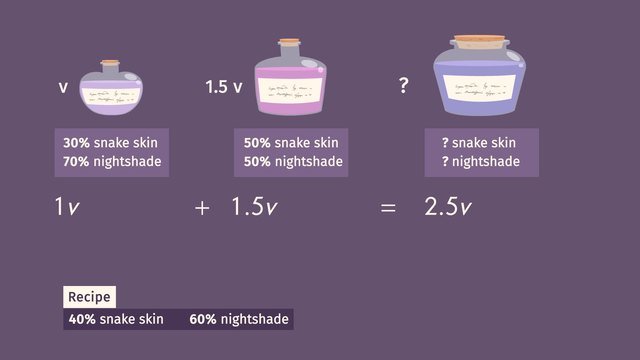# Mixture Problems Involving PercentRating

Ø 5.0 / 1 ratings

The authorsTeam Digital

## Basics on the topicMixture Problems Involving Percent

After this lesson, you will be able to write and use algebraic equations to solve percent word problems related to mixtures.

The lesson begins with setting up algebraic equations related to mixtures. It leads you to the formula %(Volume 1) + %(Volume 2) = Final %(Total Volume). It concludes with finding the percent of the mixture using the expression, [Final %(Total Volume)]/ Total Volume.

Learn about mixture word problems involving percentages by helping Wanda make a potion to replenish the trees.

This video includes key concepts, notation, and vocabulary such as the formula, %(Volume 1) + %(Volume 2) = Final %(Total Volume) and finding the percent of the resulting mixture using the expression, [Final %(Total Volume)]/ Total Volume.

Before watching this video, you should already be familiar with the formulas Quantity= Percent x Whole, Whole Quantity = Part + Part, and how to solve for the one of the missing values given two of the three (quantity, percent or, whole). You should also be able to do fraction computations and convert percents into fractions and decimals (and vice versa).

After watching this video, you will be prepared to learn more complicated mixture problems involving percentages.

Common Core Standard(s) in focus: 7.RP.A.2c, 7.RP.A.3, 7.EE.B.3 A video intended for math students in the 7th grade Recommended for students who are 12 - 13 years old